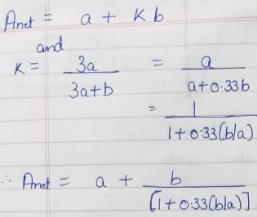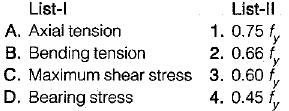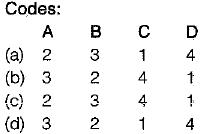Courses

# Tension Members - 2

## 10 Questions MCQ Test Mock Test Series for Civil Engineering (CE) GATE 2020 | Tension Members - 2

Description
This mock test of Tension Members - 2 for Civil Engineering (CE) helps you for every Civil Engineering (CE) entrance exam. This contains 10 Multiple Choice Questions for Civil Engineering (CE) Tension Members - 2 (mcq) to study with solutions a complete question bank. The solved questions answers in this Tension Members - 2 quiz give you a good mix of easy questions and tough questions. Civil Engineering (CE) students definitely take this Tension Members - 2 exercise for a better result in the exam. You can find other Tension Members - 2 extra questions, long questions & short questions for Civil Engineering (CE) on EduRev as well by searching above.
QUESTION: 1

### In case of single angles in tension connected by one leg only, the net effective area as per IS : 800 is Where a is net sectional area of connected leg and b is area of the outstanding leg.

Solution:QUESTION: 2

Solution:
QUESTION: 3

### Consider the following statements: Lug angles are used to 1. increase the lengths of the end connections of angle section. 2. decrease the lengths of the end connections of angle section. 3. increase the lengths of the end connections of channel section. 4. decrease the lengths of the end connections of channel section. Which of these statements are correct?

Solution:

Lug angles are sometimes used to reduce the length of the connections. However their main purpose is to accommodate more number of rivets so that size of the gusset plate may be reduced.

QUESTION: 4

Failure of tension member is considered when either of following statement is true
1. Excessive elongation in member.
2. Rupture of critical section.
3. Buckling of member.
4. Shear block failure of end connection.

Solution:

The three criterion for failure has been considered in new code. Buckling takes place in compression members and not in tension members.

QUESTION: 5

In a tension member if one or more than one rivet hole are off the line, the failure of the member depends upon

Solution:
QUESTION: 6

Tacking rivets in tension members, are provided at a pitch in line not exceeding

Solution:
QUESTION: 7

For double angles carrying tension placed back to back and connected to either side of the gusset plate, the sectional area of the section, is equal to gross sectional area of

Solution:
QUESTION: 8

Match List-I (Type of stress) with List-ll (Allowable value of stress) and select the correct answer using the codes given below the lists:
(fy = minimum yield stress of steel)Solution:
QUESTION: 9

For a single section used as a tension member, the given area is assumed

Solution:
QUESTION: 10

A tension member, if subjected to possible reversal of stress due to wind or earthquake the slenderness ratio of the member should not exceed

Solution: